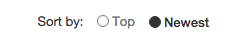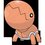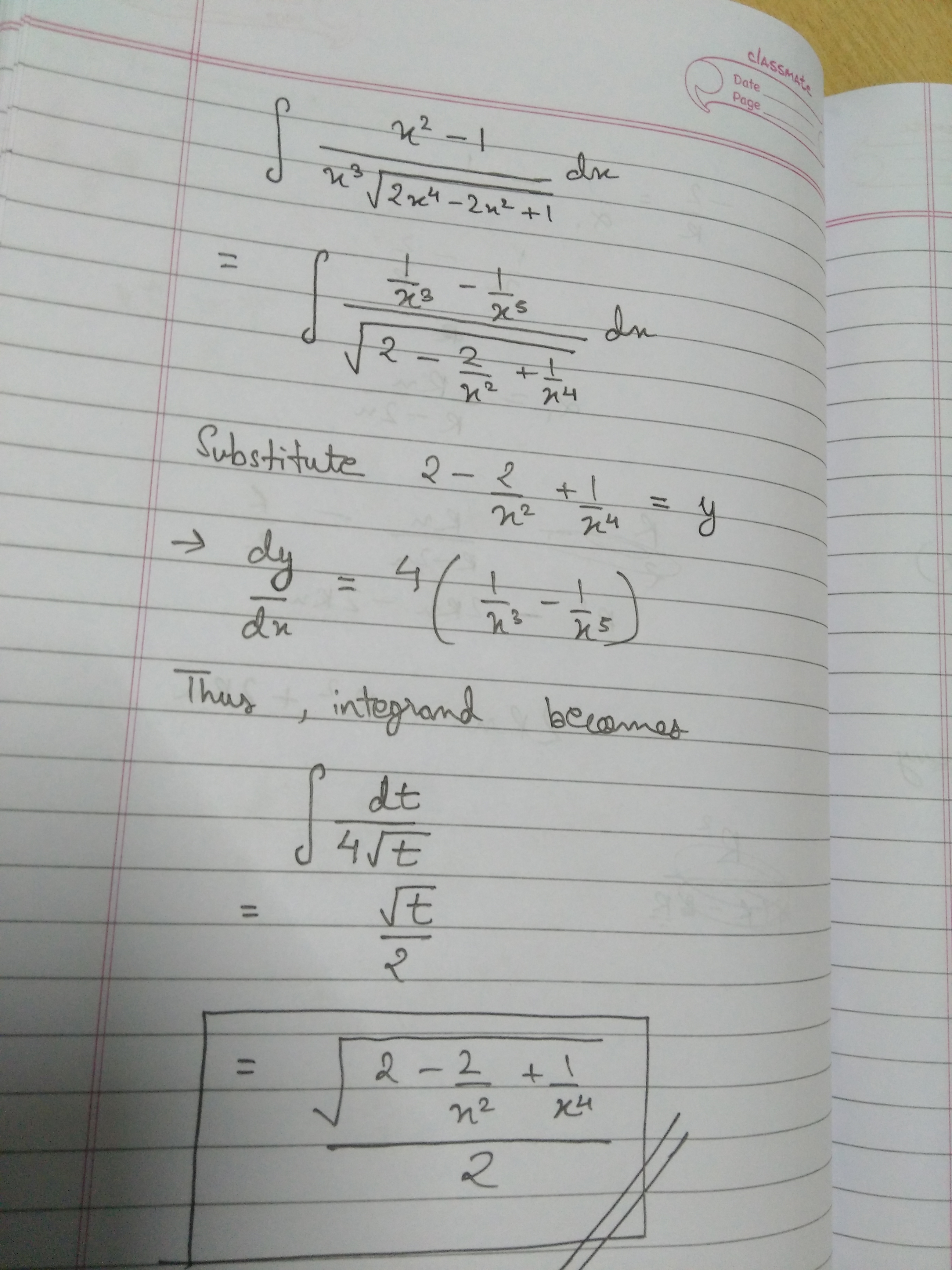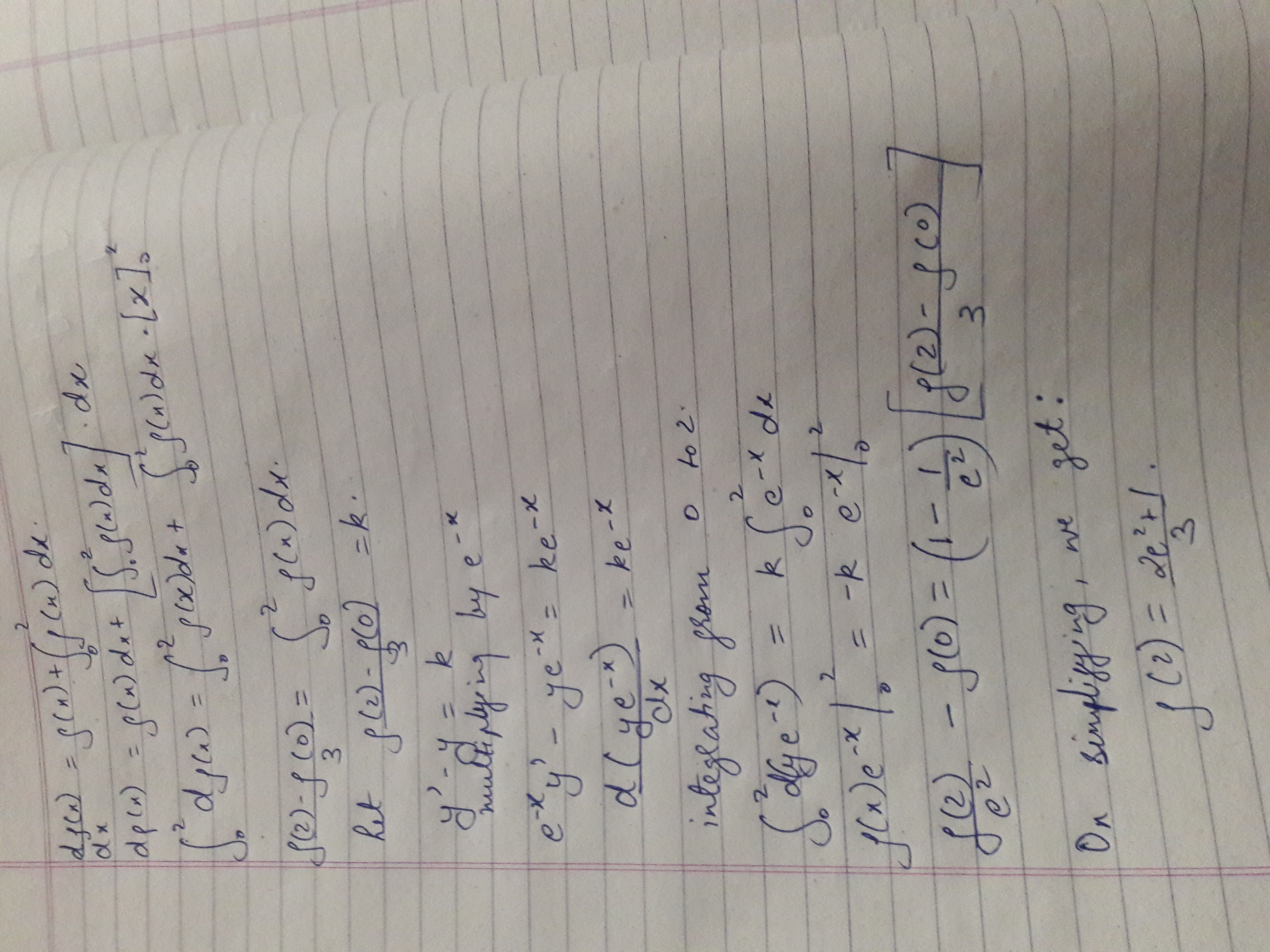# Brilliant Sub Junior Calculus Contest (Season-1) Note-2

This is the second note to the season of Brilliant Sub Junior Calculus Contest (Season-1).

Click here for First Note (if you want to visit it)

Hi everyone! This is the first season of Brilliant Sub Junior Calculus Contest. This contest is for beginners or intermediate ones who want to sharpen their skills of problem-solving in overall calculus.

The aim of this contest is to improve the skill of in the computation in all sorts of problem (of basic level) in calculus like integrals (both definite and indefinite), differentiation, limits, ecetra by learning from each other and of course to have fun!

Eligibility:- People should fulfill either of the 2 following

• 17 years or below

• Level 4 or below in Calculus

Eligible people here may participate in this contest.

The rules are as follows:

• I will start by posting the first problem. If there is a user solves it, then they must post a new one.

• You may only post a solution of the problem below the thread of problem and post your proposed problem in a new thread. Put them separately.

• Only make substantial comment that will contribute to the discussion.

• Make sure you know how to solve your own problem before posting it in case there is no one can answer it within 48 hours, then you must post the solution and you have a right to post another problem.

• If the one who solves the last problem does not post his/her own problem after solving it within a day, then the one who has a right to post a problem is the last solver before him/her.

• The scope of questions is only computation of basic level problems in calculus.

• It is NOT compulsory to post original problems. But make sure it has not been posted on brilliant.

• You are also NOT allowed to post a solution using a contour integration or residue method.

Answer shouldn't contain any Special Function.

Please post your solution and your proposed problem in a single new thread.

Format your post is as follows:

 1 2 3 4 5 6 7 **SOLUTION OF PROBLEM xxx (number of problem) :** **[Post your solution here]** **PROBLEM xxx (number of problem) :** **[Post your problem here]** 

The comments will be easiest to follow if you sort by "Newest":Note by Akshay Yadav
5 years, 2 months ago

This discussion board is a place to discuss our Daily Challenges and the math and science related to those challenges. Explanations are more than just a solution — they should explain the steps and thinking strategies that you used to obtain the solution. Comments should further the discussion of math and science.

When posting on Brilliant:

• Use the emojis to react to an explanation, whether you're congratulating a job well done , or just really confused .
• Ask specific questions about the challenge or the steps in somebody's explanation. Well-posed questions can add a lot to the discussion, but posting "I don't understand!" doesn't help anyone.
• Try to contribute something new to the discussion, whether it is an extension, generalization or other idea related to the challenge.
• Stay on topic — we're all here to learn more about math and science, not to hear about your favorite get-rich-quick scheme or current world events.

MarkdownAppears as
*italics* or _italics_ italics
**bold** or __bold__ bold
- bulleted- list
• bulleted
• list
1. numbered2. list
1. numbered
2. list
Note: you must add a full line of space before and after lists for them to show up correctly
paragraph 1paragraph 2

paragraph 1

paragraph 2

[example link](https://brilliant.org)example link
> This is a quote
This is a quote
    # I indented these lines
# 4 spaces, and now they show
# up as a code block.

print "hello world"
# I indented these lines
# 4 spaces, and now they show
# up as a code block.

print "hello world"
MathAppears as
Remember to wrap math in $$ ... $$ or $ ... $ to ensure proper formatting.
2 \times 3 $2 \times 3$
2^{34} $2^{34}$
a_{i-1} $a_{i-1}$
\frac{2}{3} $\frac{2}{3}$
\sqrt{2} $\sqrt{2}$
\sum_{i=1}^3 $\sum_{i=1}^3$
\sin \theta $\sin \theta$
\boxed{123} $\boxed{123}$

## Comments

Sort by:

Top Newest

Problem 23 $\large\int\dfrac{x^2-1}{x^3\sqrt{2x^4-2x^2+1}}\,dx=~?$

This problem has been solved by Harsh Shrivastava.

- 5 years, 2 months ago

Log in to reply- 5 years, 2 months ago

Log in to reply

A similar problem like this came in JEE Mains 2016! I just saw the question paper and recalled this problem ;)

- 5 years, 2 months ago

Log in to reply

Yeah.

- 5 years, 2 months ago

Log in to reply

Problem 25

Prove that:

$\dfrac{5050\displaystyle \int_0^1(1-x^{50})^{100}\,\mathrm{d}x}\int_0^1(1-x^{50})^{101}\,\mathrm{d}x}=\boxed{5051$

This problem has been solved by Aditya Sharma.

- 5 years, 2 months ago

Log in to reply

Solution without using Beta function: $\dfrac{5050\overbrace\int_0^1(1-x^{50})^{100}\,\mathrm{d}x}^{\color{#D61F06}{I_1}}}{\underbrace\int_0^1(1-x^{50})^{101}\,\mathrm{d}x}_{\color{#D61F06}{I_2}}$

Applying IBP on $I_2$ $I_2=x(1-x^{50})^{101}|_0^1+5050\displaystyle \int_0^1 x^{50}(1-x^{50})^{100}\,\mathrm{d}x$ $=0+5050\displaystyle \int_0^1(\color{#20A900}{1}-(\color{#20A900}{1}- x^{50}))(1-x^{50})^{100}\,\mathrm{d}x$

$=5050\left(\displaystyle \int_0^1(1-x^{50})^{100}\,\mathrm{d}x-\displaystyle \int_0^1(1-x^{50})^{101}\,\mathrm{d}x\right)$

$\implies I_2=5050(I_1-I_2)$

Rearranging we get:

$\dfrac{5050I_1}{I_2}=\boxed{5051}$

- 5 years, 2 months ago

Log in to reply

Both methods are same. The proof of Gamma functional equation uses IBP, so both methods are essentially the same.

- 5 years, 2 months ago

Log in to reply

Problem 25 : Let us first evaluate the numerator & denote it as $I_1$

Note : $\mathbf{Beta}$ Function is used which is as follows just to recall :

$\large \int_{0}^{1} x^{m-1}(1-x)^{n-1}=\beta(m,n)=\frac{\Gamma(m)\Gamma(n)}{\Gamma(m+n)}=\frac{(m-1)!(n-1)!}{(m+n-1)!}$ with m,n > 0.

Let $1-x^{50}=t$

$-50x^{49}dx=dt$

$x^{49} = (1-t)^{\frac{49}{50}}$ & $dx=\frac{dt}{-50(1-t)^{\frac{49}{50}}}$

The integral is changed to :

$I_1=-\frac{1}{50}\int_{1}^{0} t^{100}(1-t)^{-\frac{49}{50}}dt$

$50I_1=\int_{0}^{1} t^{101-1} (1-t)^{\frac{1}{50}-1}dt$

$50I_1=\beta(101,\frac{1}{50}=\frac{\Gamma(101)\Gamma(\frac{1}{50})}{\Gamma(101+\frac{1}{50})}$

Similarly the denominator just differs in case of the exponent of t, denote the denominator by $I_2$ so we have ,

$50I_2=\beta(102,\frac{1}{50})=\frac{\Gamma(102)\Gamma(\frac{1}{50})}{\Gamma(102+\frac{1}{50})}$

$50I_2=\frac{101\Gamma(101)\Gamma(\frac{1}{50})}{\Gamma(+[101+\frac{1}{50}])}$

Since we have $\Gamma(n+1)=n\Gamma(n)$ so ,

$50I_2= \frac{101}{101+\frac{1}{50}}\frac{\Gamma(101)\Gamma(\frac{1}{50})}{\Gamma(101+\frac{1}{50})}$

$50I_2=\frac{101.50}{5051}.50I_1$

$5051=\frac{5050 . I_1}{I_2}$

Therefore ,

$\large \frac{5050.\int_{0}^{1}(1-x^{50})^{100}dx}{\int_{0}^{1}(1-x^{50})^{101}dx} = 5051$ $\large \boxed{Proved}$

- 5 years, 2 months ago

Log in to reply

$\large \mathbf{Problem}$ $\large \mathfrak{27}$

Prove that : $\large \int_{0}^{\pi} \frac{xdx}{1+cos(\alpha)sin(x)} = \frac{\alpha\pi}{sin(\alpha)}$

- 5 years, 2 months ago

Log in to reply

$\large I=\int_{0}^{\pi} \frac{xdx}{1+\cos(\alpha)\sin(x)}$

Using $\int_a^b f(x)dx=\int_a^b f(a+b-x) dx$ and adding both integrals and simplifying gives:

$I=\dfrac{\pi}{2}\left(\int_0^{\pi}\dfrac{dx}{1+(\cos \alpha)( \sin x)}\right)$ Using $\sin x=\dfrac{2\tan^2 \frac x2}{1+\tan^2\frac x2}$ and substituting $\tan^2 \frac x2=t$ such that $\dfrac{(1+\tan^2 \frac x2 )dx}{2}=dt$ .

$I={\pi}\int_{0}^{\infty}\left(\dfrac{dt}{1+t^2+2\cos \alpha t}\right)$

$I={\pi}\int_{0}^{\infty}\left(\dfrac{dt}{(t+\cos \alpha )^2+\sin^2 \alpha }\right)$

Using $\int \dfrac{dx}{x^2+a^2}=\frac 1a(\tan^{-1} \frac xa)$ .

$=\dfrac{\pi}{\sin \alpha}\left(\tan^{-1}\left(\dfrac{t+\cos \alpha }{\sin \alpha}\right)\right)|_0^{\infty}$

$=\dfrac{\pi}{\sin \alpha}(\dfrac{\pi}{2}-\underbrace{\tan^{-1} \left(\cot \alpha\right)}_{\dfrac{\pi}{2}-\alpha})$

$\large \boxed{=\dfrac{\pi\alpha}{\sin \alpha}}$

- 5 years, 2 months ago

Log in to reply

Problem 24:

Evaluate $\displaystyle \int \dfrac{dx}{(\ln^{3} (\tan x))(\sin 2x)}$

This problem was solved by Rishabh Cool.

- 5 years, 2 months ago

Log in to reply

Solution to Problem 24

Substitute $\ln \tan x=t$ such that $\dfrac{dx}{\sin 2x}=\dfrac{dt}{2}$. Integral transforms to: $\int \dfrac{dt}{2 t^3}$ $=\dfrac{-1}{ 4t^2}=\dfrac{-1}{4\ln^2 \tan x}+C$

- 5 years, 2 months ago

Log in to reply

$\mathbf{Problem}$ $\mathbf{26}$

Let $f(x)$ be a function satisfying $f(0)=2,f'(0)=3,f''(x)=f(x)$ , then prove that :

$f(4) = \frac{5(e^8-1)}{2e^4}$

- 5 years, 2 months ago

Log in to reply

Let us consider a polynomial $f(x) = a + bx + cx^2 + dx^3 + ex^4 + fx^5 + gx^6 + .......$.

$f'(x) = b + 2cx + 3dx^2 + 4ex^3 + 5fx^4 +6gx^5 + 7hx^6 + .......$.

$f''(x) = 2c + 6dx + 12ex^2 + 20fx^3 + 30gx^4 + 42hx^5 + .......$.

Since , $f(0) = 2 \implies a=2$ and $f'(0) = 3 \implies b=3$.

On comparing coefficient of $x^n$ in equation $f(x) = f''(x)$

$c=1 , d= \frac{1}{2} , e =\frac{1}{12} , f=\frac{1}{40} , g=\frac{1}{360} , h =\frac{1}{1680}$

We have , $f(4) = 2 + 12 + 16 +\frac{4^3}{2}+\frac{4^4}{12} +\frac{4^5}{40} + \frac{4^6}{360}$.

Or $f(4) = \displaystyle \sum_{n=1}^\infty \frac{ 4^n ( (-1)^n + 5 ) }{ 8(n-1)!} = \displaystyle \sum_{n=1}^\infty \frac{ 4^n (-1)^n }{ 8(n-1)!} + \frac{5}{2} \displaystyle \sum_{n=1}^\infty \frac{ 4^{(n-1)} }{ (n-1)!} = \frac{1}{8} \frac{-4}{e^4} + \frac{5}{2} e^4 = \boxed{ \frac{ 5e^8 - 1}{2e^4} }$

- 5 years, 2 months ago

Log in to reply

The problem is of a book named "Integral calculus by Amit M Agaarwal" The solution he gave I am just posting it here.Now I am finding the mistake in his or sachin's solution.

$f''(x)=f(x)\implies 2f'(x)f''(x)=2f(x)f'(x)\implies d({f'(x)}^2) = d({f(x)}^2)$

$\implies {f'(x)}^2 = {f(x)}^2 + C$

Utilising the conditions for $f(0) = 2 , f'(0) = 3$ we get $C=5$

We have $f'(x) = \sqrt{5+{f(x)}^2}$

$\int dx = \int \frac{d({f(x)})}{\sqrt{5+{f(x)}^2}}$

$x + C_1 = log|f(x) + \sqrt{5+{f(x)}^2}|$

again by the conditions putting x=0 we get ,

$C_1=log5$

So, $x=log\frac{|f(x) + \sqrt{5+{f(x)}^2}|}{5}$ ..................................... (1)

Observe that , $log\frac{|f(x) + \sqrt{5+{f(x)}^2}|}{5} = log\frac{5}{|\sqrt{5+{f(x)}^2}-f(x)|}$

From (1) ,

$5e^x = f(x) + \sqrt{5+{f(x)}^2}$ & similarly $5e^{-x}=\sqrt{5+{f(x)}^2}-f(x)$

Extracting f(x) we get ,

$2f(x) = 5(e^x-e^{-x})\implies f(x) = \frac{5(e^x-e^{-x})}{2}$

$\large\boxed{ f(4) = \frac{5(e^4-e^{-4})}{2} = \frac{5(e^8-1)}{2e^4}}$

- 5 years, 2 months ago

Log in to reply

The problem is incorrect. If a polynomial $f(x)$ satisfies $f''(x) = f(x)$, then, even without solving the differential equation, the number of roots of $f(x)$ equals the number of roots of $f''(x)$, which is a contradiction. So it must be stated that $f$ is a function, not a polynomial function.

- 5 years, 2 months ago

Log in to reply

This is correct ^. In reality the function is exponential.

- 5 years, 2 months ago

Log in to reply

Problem 28 $\large\mathfrak{S}=\sum_{n=1}^x\left(\dfrac{1}{n(n+1)(n+2)\cdots(n+p)}\right)$

$\dfrac{\displaystyle\int_0^{2016}\left(\left(\frac1{p^2(p-1)!}-\mathfrak S\right)\dfrac{p(x+p)!}{x!}\right)dx}{\displaystyle\int_0^{2}\left(\left(\frac1{p^2(p-1)!}-\mathfrak S\right)\dfrac{p(x+p)!}{(x-2)!}\right)dx}= \color{#3D99F6}{\varphi}$

Find $\dfrac{\sqrt{60^2-\color{#3D99F6}{\varphi}}}{3!}$.

**Original

- 5 years, 2 months ago

Log in to reply

Solution to Problem 28:

There is a quick technique to solve the summations of this kind..... Slash of the first term and write down the other terms as it is in deno. and multiply by the number of remaining terms in the denominator (here 'p') now multiply this result with (-1) and add a constant C so the result till now is : $\\ S=C-\dfrac{1}{p(x+1)(x+2)\cdots(x+p)} \\$

When $x=1, \ S=\dfrac{1}{(p+1)!} \ also \ S=C-\dfrac{1}{p(p+1)!} \\$ This gives $C=\dfrac{1}{p.p!}$

In both the integrals $\dfrac{1}{p^2(p-1)!}-S$ is equivalent to $C-(C-\dfrac{1}{p(x+1)(x+2)\cdots(x+p)}) \\$ which is equivalent to $\dfrac{x!}{p(x+p)!}$

Now the integral in numerator turns into

$\displaystyle \int_0^{2016} dx$ and that in denominator turns into

$\displaystyle \int_0^{2} (x)(x-1)dx$

Thus, $\varphi=3024$ and answer is 4.

- 5 years, 2 months ago

Log in to reply

Problem 30

$\large\displaystyle \int \dfrac{\mathrm{d}x}{x^{11}\sqrt{1+x^4}}=\ ?$

- 5 years, 2 months ago

Log in to reply

$I = \displaystyle \int \frac{\mathrm{d}x}{x^{11}\sqrt{1+x^4}}=$

Let $\frac{1}{x^4} = t$

So , $I = - \frac{1}{4} \displaystyle \int \dfrac{t^2 \mathrm{d}t}{\sqrt{1+t}}$

Adding and subtracting 1 in numerator , And integrating ,

We have $I = - \frac{1}{4} {[ \frac{2}{15} (t+1)^{3/2} ( 3t -7) + 2\sqrt{t+1} ]} + C$

After putting the value of t in terms of x and simplifying , Now , $I = - \frac{ \sqrt{x^4 + 1}( 8x^8 - 4x^4 + 3)}{30x^{10}} + C$

- 5 years, 2 months ago

Log in to reply

Problem 29:

Prove that:

$\displaystyle \lim_{x\to 0} \dfrac{24}{x^3} \displaystyle \int_0^x \dfrac{t \ln(1+t)}{t^4+4} dt =2$

- 5 years, 2 months ago

Log in to reply

Applying LHopital:

$\lim_{x\to 0}\dfrac{8x\ln(1+x)}{x^2(x^4+4)}$

$=8\lim_{x\to 0}\dfrac{\ln(1+x)}{x(4)}$

$\text{Using} \lim_{x\to 0}\dfrac{\ln(1+x)}{x}=1$

$=\boxed 2$

- 5 years, 2 months ago

Log in to reply

Problem 31

If $I_{1} = \displaystyle \int_0^{\frac{\pi}{2} } f( \sin 2x) \sin x \mathrm{d}x$ and $I_{2} = \displaystyle \int_0^{\frac{\pi}{4} } f( \cos 2x) \cos x \mathrm{d}x$ , then find $\frac{I_{1}}{I_{2}}$ ?

- 5 years, 2 months ago

Log in to reply

$I_{1} = \displaystyle \int_{0}^{\dfrac{\pi}{2}} f(\sin 2x)\sin x dx$
Using the property,
$\displaystyle \int_{0}^{2a}f(x)dx = \int_{0}^{a}f(x)+f(2a-x)dx$
$I_{1} = \displaystyle \int_{0}^{\dfrac{\pi}{4}} f(\sin 2x)(\cos x + \sin x ) dx$
In $I_{2}$ ,
Use $\displaystyle \int_{a}^{b}f(x)dx = \int_{a}^{b} f(a+b-x)dx$
$\therefore I_{2} = \displaystyle \int_{0}^{\dfrac{\pi}{4}} f(\sin 2x)\cos\left( \dfrac{\pi}{4} - x\right) dx$
$\therefore I_{2} = \dfrac{1}{\sqrt{2}}\cdot \displaystyle \int_{0}^{\dfrac{\pi}{4}} f(\sin 2x)(\cos x + \sin x) dx = \dfrac{I_{1}}{\sqrt{2}}$
$\dfrac{I_{1}}{I_{2}} = \sqrt{2}$

- 5 years, 2 months ago

Log in to reply

Let 's define the term,
$I_{n} = \displaystyle \int_{0}^{\infty} \dfrac{\arctan\left(\frac{2x}{1}\right) + \arctan\left(\frac{3x}{2}\right) + \ldots \arctan\left(\frac{(n+1)x}{n}\right) - n\arctan(x)}{x} dx$ for integer n.

Find $I_{20} - I_{6}$

- 5 years, 2 months ago

Log in to reply

PROBLEM 33 $\displaystyle \int \sqrt{\tan x} \ dx$

The answer is very long so be patient.!

- 5 years, 2 months ago

Log in to reply

$\tan x = t^{2}$
$dx = \dfrac{2t}{1+t^{4}}dt$
$I = \displaystyle \int \dfrac{2t^{2}}{1+t^{4}}dt$
$I = \displaystyle \int \dfrac{t^{2}-1}{1+t^{4}}dt + \int \dfrac{t^{2}+1}{1+t^{4}}dt$

$I = \displaystyle \int \dfrac{1-\frac{1}{t^{2}}}{t^{2} + \dfrac{1}{t^{2}}}dt + \int \dfrac{1+\frac{1}{t^{2}}}{t^{2} + \dfrac{1}{t^{2}}}dt$

$I = \displaystyle \int \dfrac{1-\frac{1}{t^{2}}}{\left(t+\frac{1}{t} \right)^{2} - 2}dt + \int \dfrac{1+\frac{1}{t^{2}}}{\left(t-\dfrac{1}{t}\right)^{2} + 2 }dt$
$I = \displaystyle \int \dfrac{du}{u^{2} - (\sqrt{2})^{2}} + \int \dfrac{dv}{v^{2}+(\sqrt{2})^{2}}$
$I =\dfrac{1}{2\sqrt{2}}\ln\left(\dfrac{u-\sqrt{2}}{u+\sqrt{2}}\right) + \dfrac{1}{\sqrt{2}}\cdot \arctan\left(\dfrac{v}{\sqrt{2}}\right)$

$I = \dfrac{1}{2\sqrt{2}}\ln\left(\dfrac{t+\frac{1}{t}-\sqrt{2}}{t+\frac{1}{t}+\sqrt{2}}\right)+ \dfrac{1}{\sqrt{2}}\cdot \arctan\left(\dfrac{t-\frac{1}{t}}{\sqrt{2}}\right)$

$I = \dfrac{1}{2\sqrt{2}}\ln\left(\dfrac{\sqrt{\tan(x)} + \sqrt{\cot(x)}-\sqrt{2}}{\sqrt{\tan(x)} + \sqrt{\cot(x)}+\sqrt{2}}\right)+\dfrac{1}{\sqrt{2}}\cdot \arctan\left(\dfrac{\sqrt{\tan(x)}-\sqrt{\cot(x)}}{\sqrt{2}}\right) + c$

- 5 years, 2 months ago

Log in to reply

Will you post next problem or shall we post? You often stall the contest.

(Sorry to be rude)

- 5 years, 2 months ago

Log in to reply

You post. I have been trying to come up with a good problem, but I haven't been getting one.

- 5 years, 2 months ago

Log in to reply

Okay according to rules @Samarth Agarwal should post the next problem.

- 5 years, 2 months ago

Log in to reply

Very nice..... Pls post the next question

- 5 years, 2 months ago

Log in to reply

Problem 37

Let $f(x)$ be a differentiable function such that $\displaystyle \dfrac{d f(x) }{dx} = f(x) + \int_0^2 f(x) \, dx$ and $f(0) = \dfrac{4-e^2}3$, then prove: $f(2)=\dfrac{2e^2+1}{3}$

- 5 years, 2 months ago

Log in to reply- 5 years, 2 months ago

Log in to reply

@Rishabh Cool is this your original problem??? Btw Its really very nice

- 5 years, 2 months ago

Log in to reply

Nope... :-).... I'm not sure but it might be an IIT JEE problem.... I found this problem interesting so I found it worth sharing.

- 5 years, 2 months ago

Log in to reply

I don't have a good problem right now.... Can anybody else post the question... Thanx

- 5 years, 2 months ago

Log in to reply

Sometimes I don't get differential equations, what should I do?

- 5 years, 2 months ago

Log in to reply

Only practice helps in this topic..... And there are some standard rules which are needed to be followed

- 5 years, 2 months ago

Log in to reply

Can i post the next question?

- 5 years, 2 months ago

Log in to reply

Yeah! Anybody is welcome to do so.

- 5 years, 2 months ago

Log in to reply

Can we change the note after 40 problems as my phone has already started lagging

- 5 years, 2 months ago

Log in to reply

Same is happening with my phone too...

- 5 years, 2 months ago

Log in to reply

My tablet too is going crazy. I will change it tomorrow (even if the question number doesn't crosses 40). Till then let's hope it the question number crosses 40!

- 5 years, 2 months ago

Log in to reply

Yes if @Akshay Yadav allows

- 5 years, 2 months ago

Log in to reply

$\text{Problem 38}$What is the value of the integral given below?$\int\dfrac{\tan x}{a+b\tan^2x}dx$

- 5 years, 2 months ago

Log in to reply

My method was to take denominator as t and then apply a little partial fraction giving the same answer... :)

@Aditya Sharma you may post the next question

- 5 years, 2 months ago

Log in to reply

$\large I = \int \frac{tanx}{a+b(tan)^2x}\ = \int \frac{sin(x)cos(x)}{acos^2x+bsin^2x}$

$\text{Put } sin^2x=t$

$I = \frac{1}{2}\int \frac{sin(2x)}{a - sin^2x(a-b)} = \int \frac{dt}{2(a - t(a-b))} = \frac{1}{2(b-a)}ln|a-t(a-b)| + C$

- 5 years, 2 months ago

Log in to reply

Problem no : 32

$f(x) = 3e^{x} + \dfrac{1}{1+x^{2}}$
Let $g(x)$ be the inverse of $f(x)$.
Compute,
$\displaystyle \int_{3}^{3e - \frac{1}{2}}g(x+1)dx$

- 5 years, 2 months ago

Log in to reply

$I = \displaystyle \int_{3}^{3e - \frac{1}{2}} g(x+1) dx = \int_{4}^{3e+\frac{1}{2}} g(x)dx = \int_{f(0)}^{f(1)}g(x)dx$
Substitute, $g(x) = t \rightarrow f(g(x)) = f(t)$
But since, $f(x)$ and $g(x)$ are inverses of each other, $f(g(x))= x$
$\therefore x = f(t) \rightarrow dx = f'(t)dt$
$\therefore I = \displaystyle \int_{0}^{1} tf'(t)dt$
Integrating by parts,
$I = \left[tf(t)\right]_{0}^{1} - \displaystyle \int_{0}^{1} f(t)dt$
$I = 3e + \dfrac{1}{2} - \displaystyle \int_{0}^{1} 3e^{t} + \dfrac{1}{1+t^{2}}dt = 3e + \dfrac{1}{2} - \left[ 3e^{t} + \tan^{1}(x) \right]_{0}^{1}$
$\therefore I = 3e + \dfrac{1}{2} -3e - \dfrac{\pi}{4} + 3 + 0 = \dfrac{7}{2} - \dfrac{\pi}{4} = \dfrac{14-\pi}{4}$

- 5 years, 2 months ago

Log in to reply

PROBLEM 34:

Find the minimum value of the integral $\displaystyle \int_0^{\pi} \left(x - \pi a - \frac {b}{\pi}\cos x\right)^2 \ dx$

- 5 years, 2 months ago

Log in to reply

I had simplified the expression and then integrated it, after simplification you get-

$\displaystyle \int_0^{\pi} \left(x^2+\pi^2a^2+\frac{b^2}{\pi^2}\cos^2 (x)-2\pi ax+2ab\cos (x)-\frac{2b}{\pi}\cos (x) \right) dx$

From that you get the expression-

$\frac{3b^2+24b+6{\pi}^4a^2-6{\pi}^4a+2{\pi}^4}{6{\pi}}$

Let this equals to $y$ and then we use partial differentiation,

From that we get $a=\frac{1}{2}$ and $b=-4$.

Place the values back and then you'll get the required minimum value of the integral as,

$\boxed{\frac{\pi^3}{12}-\frac{8}{\pi}}$

- 5 years, 2 months ago

Log in to reply

PROBLEM 35:

Time for an easy problem!

Find the radius and coordinates of center of curvature of the curve

$y=(4x-x^2-3)^{\frac{1}{2}}$

at the points $x=1.2$ and $x=2$. Also what is this curve?

- 5 years, 2 months ago

Log in to reply

It is a semi circle above x axis as y is always positive with radius 1 unit and center (2,0)

- 5 years, 2 months ago

Log in to reply

I think that question is more based on co ordinate geometry as curve is just a semi circle

- 5 years, 2 months ago

Log in to reply

Wow, I had expected a fast reply but you did it extremely fast!

- 5 years, 2 months ago

Log in to reply

This is because it just requires simple co ordinate geometry

- 5 years, 2 months ago

Log in to reply

PROBLEM 36

Let $f(x)$ be a differentiable function and $f'(x)=-f(x) , f'(x) =g(x)$

Let $h(x)=[f(x)]^2+[g(x)]^2$ and h(5)=11 find h(10)

- 5 years, 2 months ago

Log in to reply

$\dfrac{f'(x)}{f(x)}=-1$

Integrate both sides to get: $f(x)=Ce^{-x}$ $\implies g(x)=f'(x)=-Ce^{-x}$ $\implies h(x)=2C^2e^{-2x}$

$\dfrac{h(10)}{h(5)}=\dfrac{e^{-20}}{e^{-10}}=e^{-10}$

$\implies h(10)=\boxed{11e^{-10}}$

Is it or not???

- 5 years, 2 months ago

Log in to reply

Absolutely correct!!! You may post the next question :)

- 5 years, 2 months ago

Log in to reply

$\mathbf{Problem} \mathfrak{39}$ Determine : $\int \frac{dx}{secx+2sinx}$

- 5 years, 2 months ago

Log in to reply

The given integral can be written as

$\displaystyle \int \dfrac{\cos x \ dx}{1+2 \sin x \cos x}$

$\displaystyle = \int \dfrac{\cos x \ dx}{(\sin x + \cos x )^2}$

$\displaystyle = \dfrac{1}{2} \int \dfrac{(\sin x + \cos x) + (\cos x - \sin x)}{(\sin x + \cos x )^2} dx$

$\displaystyle = \dfrac{1}{2} \int \dfrac{dx}{\sin x + \cos x} + \dfrac{1}{2} \int \dfrac{d(\sin x + \cos x)}{(\sin x + \cos x)^2}$

$\displaystyle = \dfrac{1}{2\sqrt{2}} \int \csc \left(x + \frac{\pi}{4}\right) dx + \dfrac{1}{2} \int \dfrac{d(\sin x + \cos x)}{(\sin x + \cos x)^2}$

$\displaystyle = -\dfrac{1}{2\sqrt{2}} \ln \left| \csc\left( x +\frac{\pi}{4}\right) + \cot \left(x+\frac{\pi}{4}\right) \right|-\dfrac{1}{2(\sin x +\cos x)} + C$

- 5 years, 2 months ago

Log in to reply

Yeah same form as @Adarsh Kumar , He just simplified the last one to $\frac{1}{2\sqrt2}\frac{1}{sin(x+\frac{\pi}{4})}$

- 5 years, 2 months ago

Log in to reply

I think @Akshay Yadav now the note should be changed and the next note will begin with problem 40 as my PC,tablet,Mobile everything is at a standstill :P

- 5 years, 2 months ago

Log in to reply

My method was a little b